# Machine Learning with Wio Terminal - Gesture Recognition

This tutorial will demonstrates how to use Wio Terminal to set up a simple gesture recognition machine learning demo with the help of TensorFlow Lite.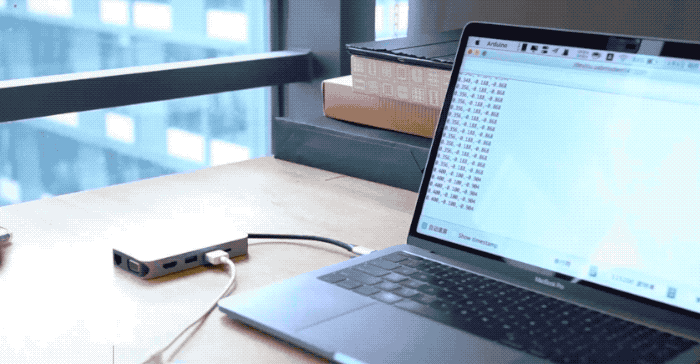This example is modified from the official demo of Arduino team together with TensorFlow Lite team. For more information and reference, please visit Get started with machine learning on Arduino. Some modifications are done to make the demo compatible with Wio Terminal.

## Features​

• Machine Learning on Wio Terminal

• Gestures Recognition

• TensorFlow Lite

## Arduino Libraries Needed​

• Install the on-board accelerator library `Seeed_Arduino_LIS3DHTR`, Please visit Wio Terminal IMU for more information.

## Training IMU data from the Wio Terminal​

Just like other machine learning project, we will obtain some sensor data to be used for training the model later. First, we will run a simple arduino program to stream sensor data from Wio Terminal.

Copy the following code and upload the code to Wio Terminal:

``#include"LIS3DHTR.h"LIS3DHTR<TwoWire> lis;const float accelerationThreshold = 2; // threshold of significant in G'sconst int numSamples = 119;int samplesRead = numSamples;void setup() {  Serial.begin(9600);  lis.begin(Wire1);  if (!lis) {    Serial.println("ERROR");    while(1);  }    lis.setOutputDataRate(LIS3DHTR_DATARATE_25HZ); //Data output rate  lis.setFullScaleRange(LIS3DHTR_RANGE_2G); //Scale range set to 2g  Serial.println("Acceleration in G's");  Serial.println("X\tY\tZ");}void loop() {  float x, y, z;    while (samplesRead == numSamples) {    x=lis.getAccelerationX();    y=lis.getAccelerationY();    z=lis.getAccelerationZ();     float aSum = fabs(x) + fabs(y) + fabs(z);      // check if it's above the threshold      if (aSum >= accelerationThreshold) {        // reset the sample read count        samplesRead = 0;        break;      }  }  while (samplesRead < numSamples) {    x=lis.getAccelerationX();    y=lis.getAccelerationY();    z=lis.getAccelerationZ();    samplesRead++;    Serial.print(x, 3);    Serial.print(',');    Serial.print(y, 3);    Serial.print(',');    Serial.print(z, 3);    Serial.println();    if (samplesRead == numSamples) {      Serial.println();    }  }}``

Or download the code here and upload the code to Wio Terminal. If you open the Serial Monitor, you should be able to see IMU data.

## Capturing Gestures Training Data​

Capture IMU data as CSV and used for TensorFlow:

• Open Serial Monitor, and pick up Wio Terminal.

• Make a punch gesture, make sure that you've triggered the capture.

• Repeat the same movement for at least 10 times, the more training data the better.

• Copy and paste the data from the Serial Monitor to a new text file called `punch.csv`.

• Clear and reset the Wio Terminal, and this time repeat all the steps above with a flex gesture and saved as `flex.csv`.

Note: If you are using Linux or Mac, you can directly output the sensor data from Wio Terminal to a `.csv` file using:

`` cat /dev/cu.usbmodem[nnnnn] > punch.csv``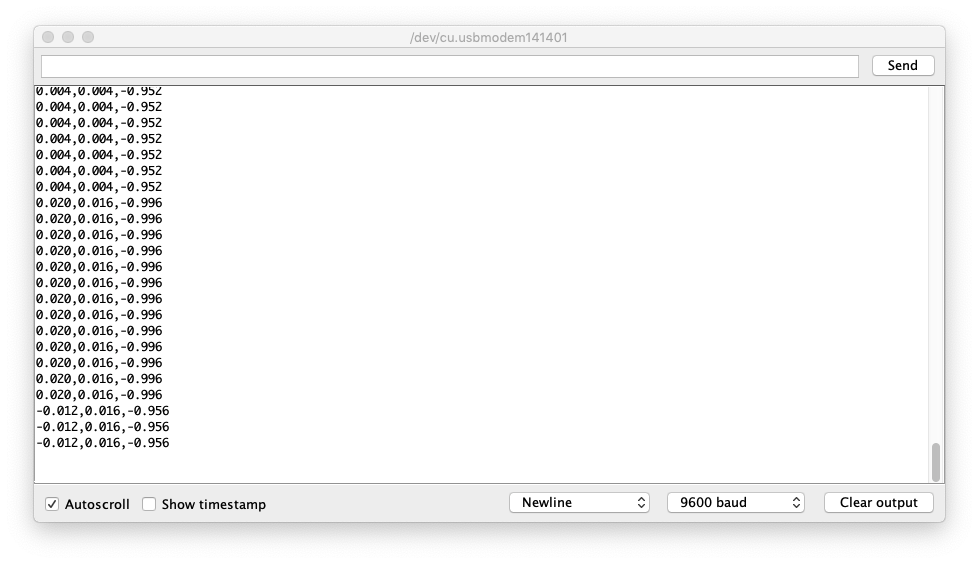## Checking the `.csv` Files​

Open the `punch.csv` and `flex.csv` and make sure that the first line of the csv files is aX,aY,aZ check below for reference: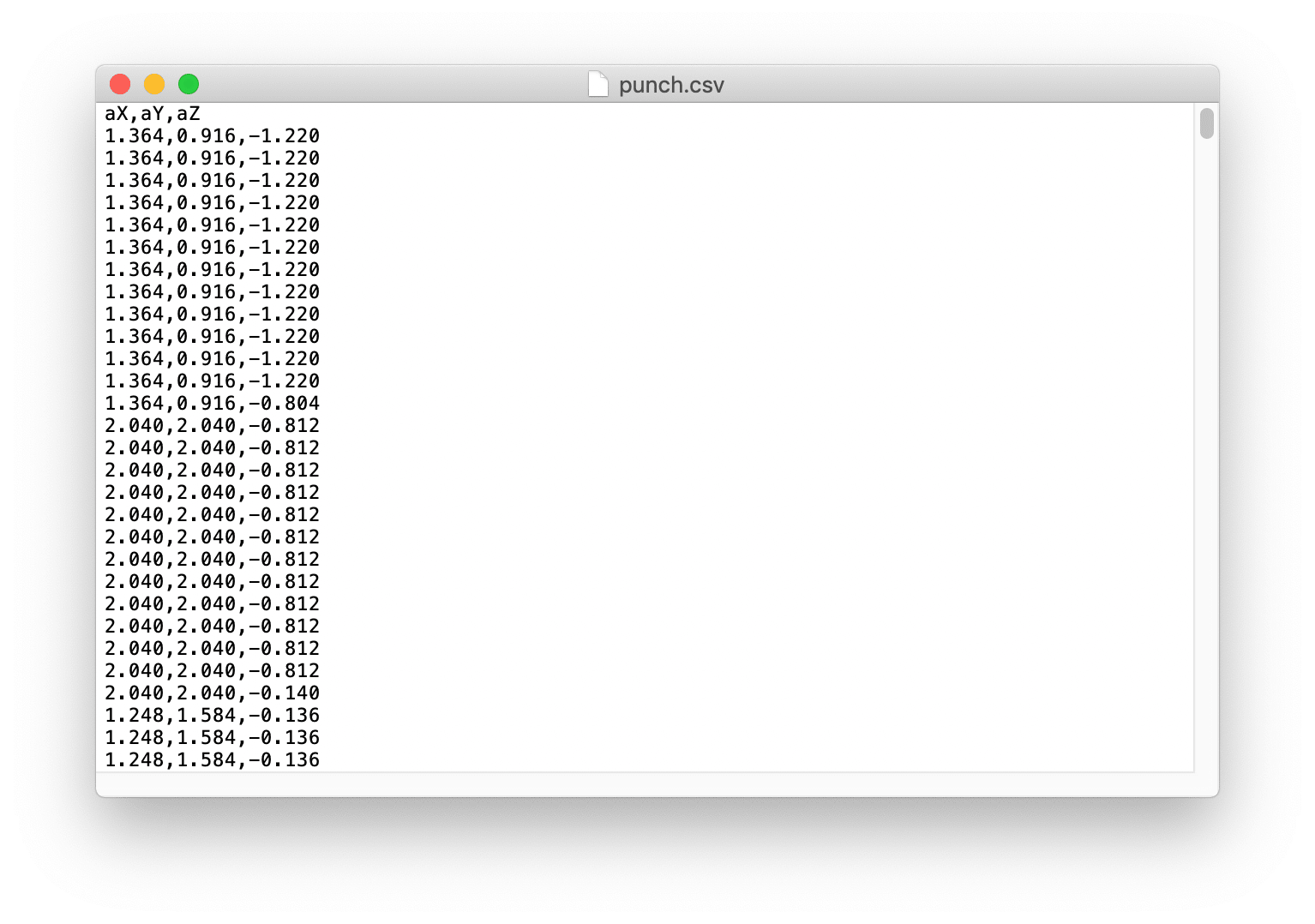## Training Data in TensorFlow​

Here we will use the Google Colab project that is created by the Arduino team. Colab provides a Jupyter notebook that allows us to run our TensorFlow training in a web browser. The colab will set up the environment, train the model and convert the model into a TensorFlow Lite mode and encode the model in an Arduino header file.

## Modifications in Google Colab Project​

Some modifications are needed in the Google Colab Project to adapt Wio Terminal:

• No changes are needed in Setup the Python Environment.

• No changes are needed in Upload Data.

• In Graph Data, comment out all the gyroscope data sections as shown: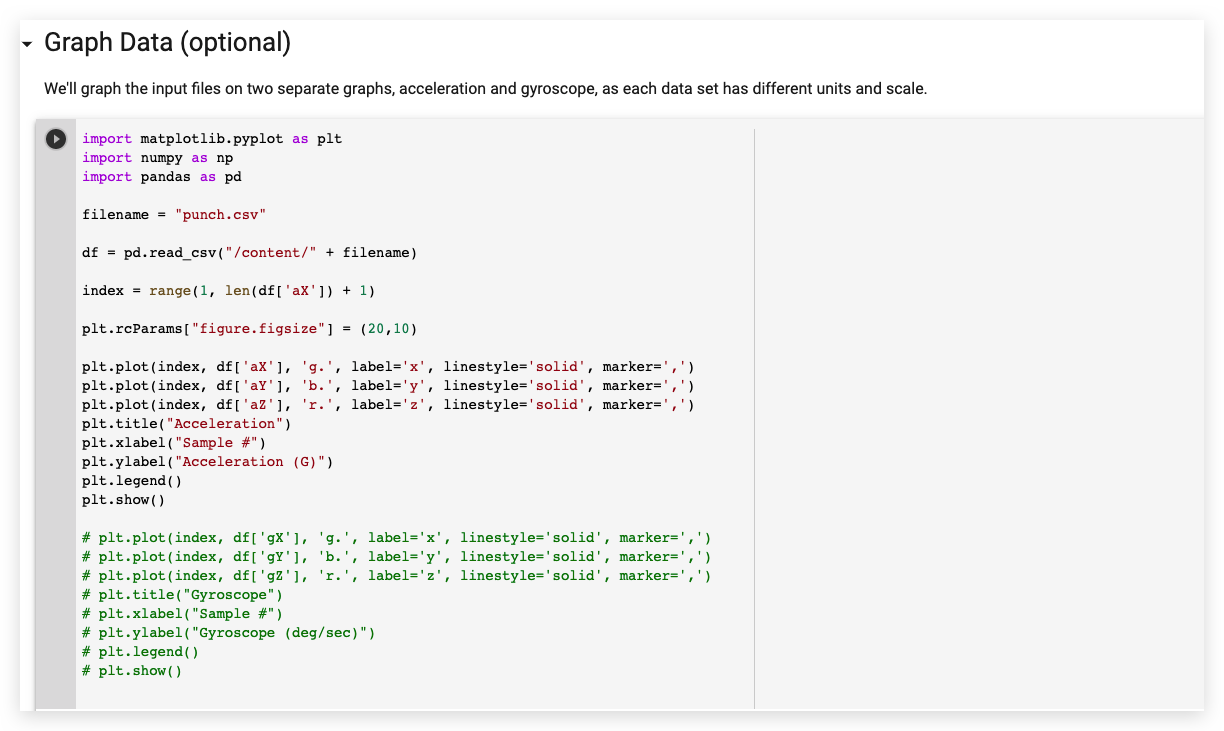• In Parse and prepare the data, comment out all the gyroscope data sections as shown: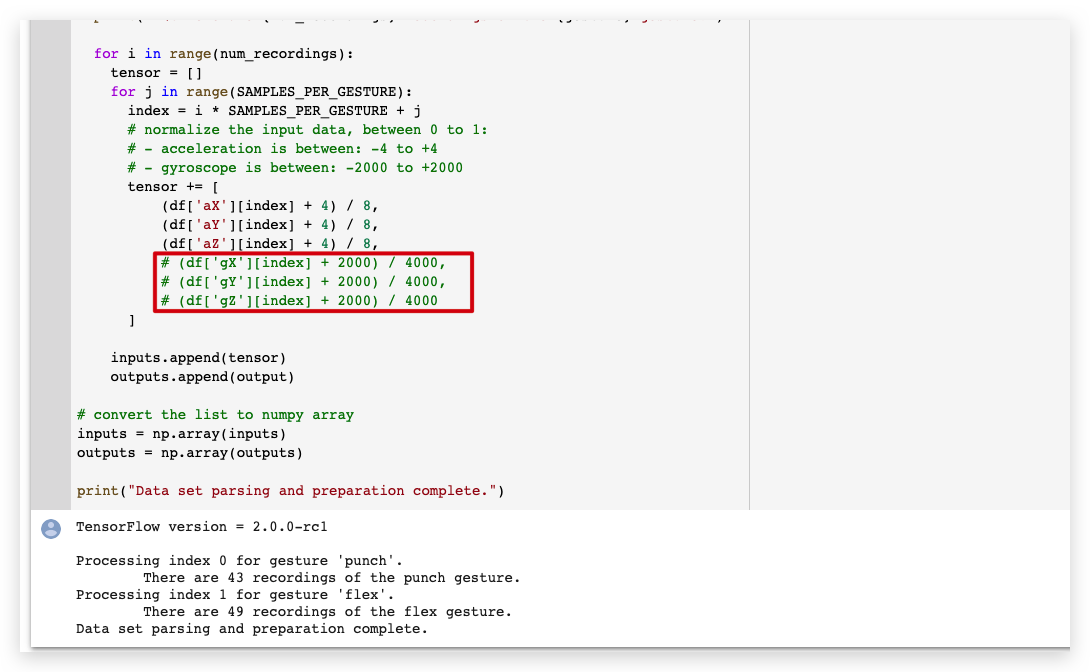• No changes are needed in Randomize and split the input and output pairs for training.

• No changes are needed in Build & Train the Model.

• No changes are needed in Graph the loss.

• No changes are needed in Graph the loss again, skipping a bit of the start.

• No changes are needed in Graph the mean absolute error.

• No changes are needed in Run with Test Data.

• No changes are needed in Convert the Trained Model to Tensor Flow Lite.

• No changes are needed in Encode the Model in an Arduino Header File.

Download the `model.h` files from the left panel.

• Once downloaded the model header files. Open IMU_Classifier.ino or copy the following code in Arduino IDE:
``/*  IMU Classifier  This example uses the on-board IMU to start reading acceleration and gyroscope  data from on-board IMU, once enough samples are read, it then uses a  TensorFlow Lite (Micro) model to try to classify the movement as a known gesture.  Note: The direct use of C/C++ pointers, namespaces, and dynamic memory is generally        discouraged in Arduino examples, and in the future the TensorFlowLite library        might change to make the sketch simpler.  The circuit:  - Arduino Nano 33 BLE or Arduino Nano 33 BLE Sense board.  Created by Don Coleman, Sandeep Mistry  Modified by Dominic Pajak, Sandeep Mistry  This example code is in the public domain.  Modified to adapt for Wio Terminal - Anson (Seeed Studio)*/#undef min#undef max#include <TensorFlowLite.h>#include <tensorflow/lite/experimental/micro/kernels/all_ops_resolver.h>#include <tensorflow/lite/experimental/micro/micro_error_reporter.h>#include <tensorflow/lite/experimental/micro/micro_interpreter.h>#include <tensorflow/lite/schema/schema_generated.h>#include <tensorflow/lite/version.h>#include "model.h"#include"LIS3DHTR.h"LIS3DHTR<TwoWire> lis;const float accelerationThreshold = 2; // threshold of significant in G'sconst int numSamples = 119;int samplesRead = numSamples;// global variables used for TensorFlow Lite (Micro)tflite::MicroErrorReporter tflErrorReporter;// pull in all the TFLM ops, you can remove this line and// only pull in the TFLM ops you need, if would like to reduce// the compiled size of the sketch.tflite::ops::micro::AllOpsResolver tflOpsResolver;const tflite::Model* tflModel = nullptr;tflite::MicroInterpreter* tflInterpreter = nullptr;TfLiteTensor* tflInputTensor = nullptr;TfLiteTensor* tflOutputTensor = nullptr;// Create a static memory buffer for TFLM, the size may need to// be adjusted based on the model you are usingconstexpr int tensorArenaSize = 8 * 1024;byte tensorArena[tensorArenaSize];// array to map gesture index to a nameconst char* GESTURES[] = {  "punch",  "flex"};#define NUM_GESTURES (sizeof(GESTURES) / sizeof(GESTURES))void setup() {  Serial.begin(9600);//  while (!Serial);  lis.begin(Wire1);    // initialize the IMU  if (!lis) {    Serial.println("Failed to initialize IMU!");    while (1);  }  // get the TFL representation of the model byte array  tflModel = tflite::GetModel(model);  if (tflModel->version() != TFLITE_SCHEMA_VERSION) {    Serial.println("Model schema mismatch!");    while (1);  }  // Create an interpreter to run the model  tflInterpreter = new tflite::MicroInterpreter(tflModel, tflOpsResolver, tensorArena, tensorArenaSize, &tflErrorReporter);  // Allocate memory for the model's input and output tensors  tflInterpreter->AllocateTensors();  // Get pointers for the model's input and output tensors  tflInputTensor = tflInterpreter->input(0);  tflOutputTensor = tflInterpreter->output(0);}void loop() {  float x, y, z;  // wait for significant motion  while (samplesRead == numSamples) {    x=lis.getAccelerationX();    y=lis.getAccelerationY();    z=lis.getAccelerationZ();    float aSum = fabs(x) + fabs(y) + fabs(z);      // check if it's above the threshold      if (aSum >= accelerationThreshold) {        // reset the sample read count        samplesRead = 0;        break;      }  }  // check if the all the required samples have been read since  // the last time the significant motion was detected  while (samplesRead < numSamples) {    // check if new acceleration AND gyroscope data is available      // read the acceleration and gyroscope data      x=lis.getAccelerationX();      y=lis.getAccelerationY();      z=lis.getAccelerationZ();      // normalize the IMU data between 0 to 1 and store in the model's      // input tensor      tflInputTensor->data.f[samplesRead * 6 + 0] = (x + 4.0) / 8.0;      tflInputTensor->data.f[samplesRead * 6 + 1] = (y + 4.0) / 8.0;      tflInputTensor->data.f[samplesRead * 6 + 2] = (z + 4.0) / 8.0;      samplesRead++;      if (samplesRead == numSamples) {        // Run inferencing        TfLiteStatus invokeStatus = tflInterpreter->Invoke();        if (invokeStatus != kTfLiteOk) {          Serial.println("Invoke failed!");          while (1);          return;        }        // Loop through the output tensor values from the model        for (int i = 0; i < NUM_GESTURES; i++) {          Serial.print(GESTURES[i]);          Serial.print(": ");          Serial.println(tflOutputTensor->data.f[i], 6);        }        Serial.println();      }  }}``
• Put the trained `model.h` header file in the same folder as the IMU_Classifier.ino file and upload the code to Wio Terminal.

• Now, open serial monitor and perform your gestures! You should see confidence of each gesture printed (0 = low confidence, 1 = high confidence).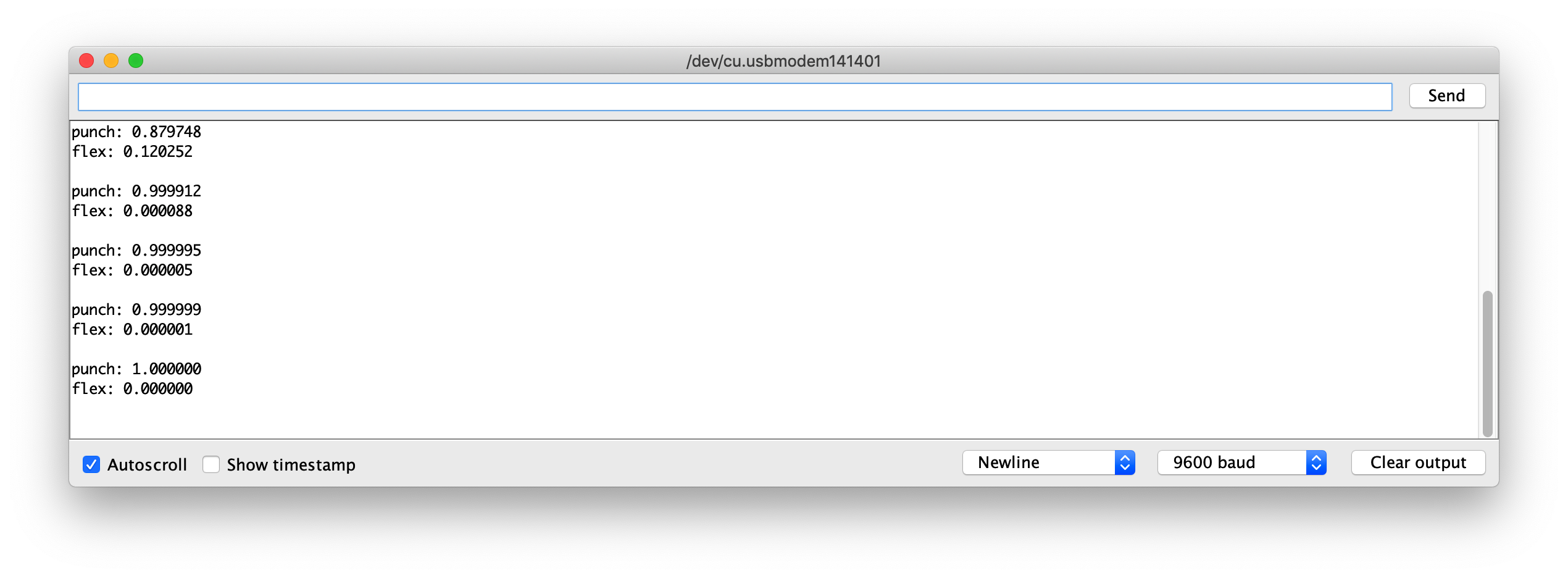## Example Model Files​

For reference, I have attached the trained-model of model.h files to download. You use this with IMU_Classifier.ino to test.

## Further Development​

For further development, more gestures can be trained on Wio Terminal via IMU, and to trigger different outputs! Explore with Machine Learning on Microcontroller with TensorFlow Lite!